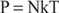## Equation of State

The internal structure of normal stars is fairly simple because only a few physical principles are involved in the determination of the structure of a gaseous object. This simplicity is summed up in a simple principle, the Russel‐Vogt theorem. For stars of like composition, the structure and observable properties depend on a single parameter such as the star's mass. Alternatively, the Russell‐Vogt theorem may be expressed as follows: The equilibrium structure of an ordinary star is determined uniquely by its mass and chemical composition. This principle or theorem is not an accident of nature, but is the direct result of how the laws of physics determine the equilibrium structure of a normal star.

This physical law relates gas pressure P in a given layer to the number density of particles N (particles/cm 3) and the temperature T at that radius from the center of the star. For a perfect gas, the equation of state sayswhere k is the Boltzman constant. Pressure is simply proportional to the number density of particles and to the temperature (expressed in Kelvin). The number of particles in a given volume, however, depends upon the chemical composition of the material, because particles are not identical. An atom of helium has four times the mass of an atom of hydrogen; thus, a given amount of helium has only one‐fourth the number of particles as an equal amount of hydrogen. The same total mass of these two elements in an identical volume therefore will have different pressures. If the pressure from helium is responsible for the balance against gravity in a stable star, then four times as much mass in the form of helium would be necessary at the same temperature to produce the same pressure as hydrogen.

Temperature is an additional factor. At low enough temperatures, atoms are electrically neutral. At higher temperatures, atoms ionize, the electron becoming free particles in addition to the nuclei. Each ionized hydrogen atom would be represented by two particles, the nucleus (proton) and a free electron, with a corresponding change in pressure compared to neutral hydrogen. Given the chemical composition of a gas and the state of ionization of the atoms, then the mean atomic mass μ of the particles may be calculated and the equation of state expressed as follows: the mass density ρ becomes P = ρkT/μ.

Back to Top
A18ACD436D5A3997E3DA2573E3FD792A